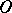# Euler straight line

(diff) ← Older revision | Latest revision (diff) | Newer revision → (diff)
The straight line passing through the pointof intersection of the altitudes of a triangle, the pointof intersection of its medians, and the centreof the circle circumscribed to it. If the Euler line passes through a vertex of the triangle, then the triangle is either isosceles or right-angled, or both right-angled and isosceles. The segments of the Euler line satisfy the relation